SCALE HARMONISATION

We now have a good knowledge of scale theory and chord theory, so our next task is key construction! This theory will allow us to understand how to work out and write our own songs using just the theory we already have learnt! It's an exciting lesson as it unlocks the door to being able to play songs by ear. It can be quite hard to grasp initially, so go through the lessons at your own pace. Have fun!

## Summary: What is key construction?

When you construct a key, you take the scale (in our case we'll be using the major scale) and build a chord from each of the 7 notes of that scale. The chord that you build can only use notes that are in that scale. For example, if you are using the key of C major, all of the chords must be built from the notes within the scale of C major. This is why the chords within the key of C major all work together and can be classed as being in the same key. Simple enough on the face of it right? Well, in the next lesson we will start putting this into practice to show you more clearly how it works!

## Summary:The 1st Degree

We start by writing out the C major scale as we are currently working out the chords within the key of C major. We then want to build a triad chord (a 3 note chord) from each degree of the scale. Taking the first degree, C, as an example, the process will be to work out the C major chord, C minor chord and C diminished chord and see which one we can use. The one we can use will only contain notes that are in the C major scale. So let's have a look: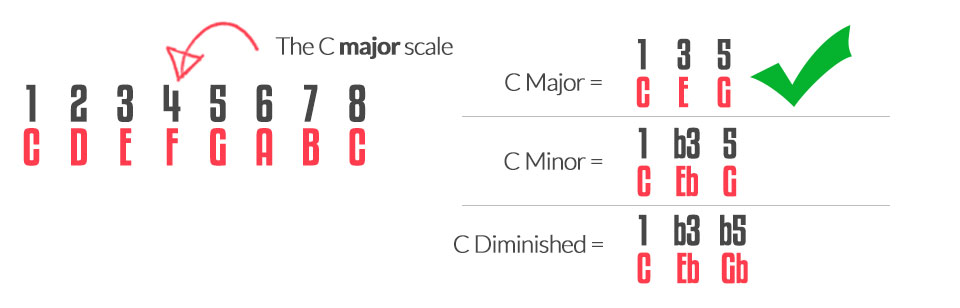So we can clearly see that the C major chord fits into the key of C major as all 3 notes fit perfectly into our the C major scale. The scale is C D E F G A B C, and in the C major chord, the notes are C, E and G. Perfect! Therefore the first chord of our key is C major.

## Summary: The 2nd Degree

We now want to move to the second degree of the major scale, which in this case is D. We need to now write down the D major scale so that we can work out the three triad chords. They look like this: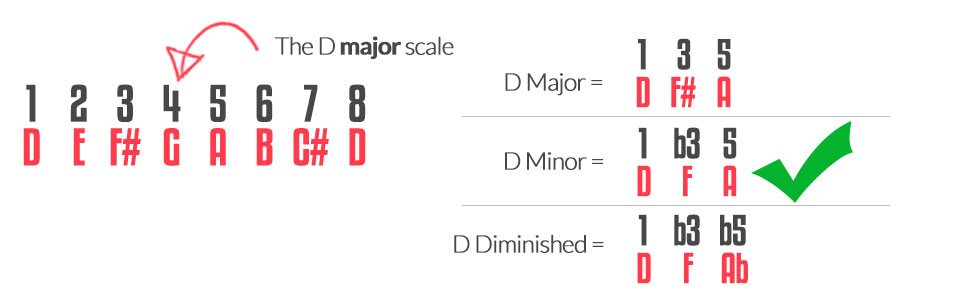We can clearly see that the D minor chord will fit into the key of C major as all 3 notes fit perfectly into our scale. The scale is C D E F G A B C, and in the D minor chord, the notes are D, F & A. Perfect! Therefore the second chord of our key is D minor.

## Summary: The 3rd Degree

We now want to move to the third degree of the major scale, which in this case is E. We need to now write down the E major scale so that we can work out the three triad chords. They look like this: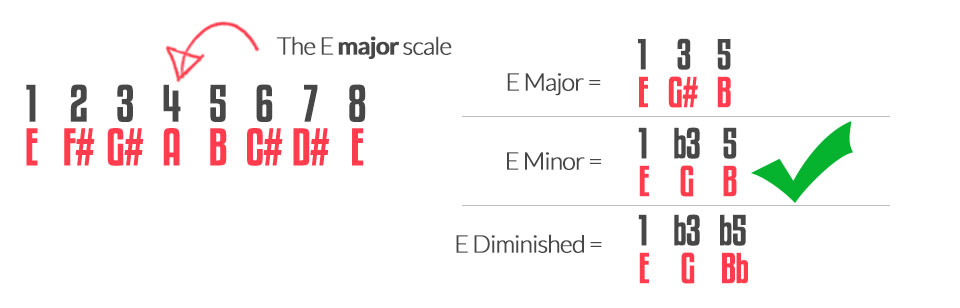So we can clearly see that the E minor chord will fit into the key of C major as all 3 notes within the chord are in the scale. The scale is C D E F G A B C, and in the E minor chord you have a E, G & B. Perfect! Therefore the third chord of our key is E minor.

## Summary: The 4th Degree

We now want to move to the fourth degree of the major scale, which in this case is F. We need to now write down the F major scale so that we can work out the three triad chords. They look like this: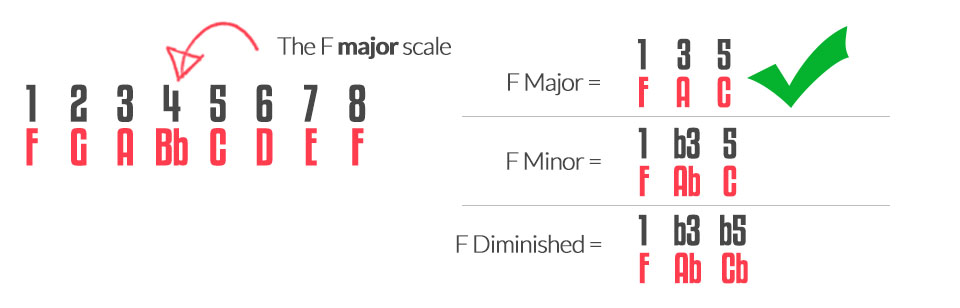So we can clearly see that the F Major chord will fit into our C major scale as all 3 notes fit perfectly into our scale. The scale is C D E F G A B C, and in the F Major chord you have a F, A & C. Perfect! Therefore the fourth chord of our key is F major.

## Summary: The 5th Degree

We now want to move to the fifth degree of the major scale, which in this case is G. We need to now write down the G major scale so that we can work out the three triad chords. They look like this: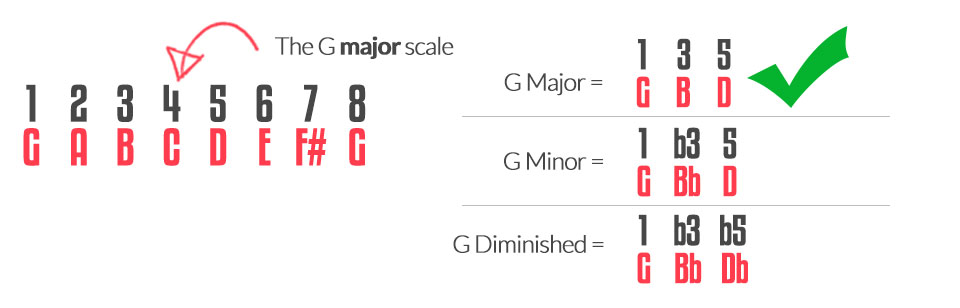So we can clearly see that the G major chord will fit into our C major scale as all 3 notes fit perfectly into our scale. The scale is C D E F G A B C, and in the G Major chord you have a G, B & D. Perfect! Therefore the fifth chord of our key is G major.

## Summary: The 6th Degree

We now want to move to the sixth degree of the major scale, which in this case is A. We need to now write down the A major scale so that we can work out the three triad chords. They look like this:So we can clearly see that the A minor chord will fit into our C major scale as all 3 notes fit perfectly into our scale. The scale is C D E F G A B C, and in the A minor chord you have a A, C & E. Perfect! Therefore the sixth chord of our key is A minor.

## Summary: The 7th Degree

We now want to move to the seventh degree of the major scale, which in this case is B. We need to now write down the B major scale so that we can work out the three triad chords. They look like this: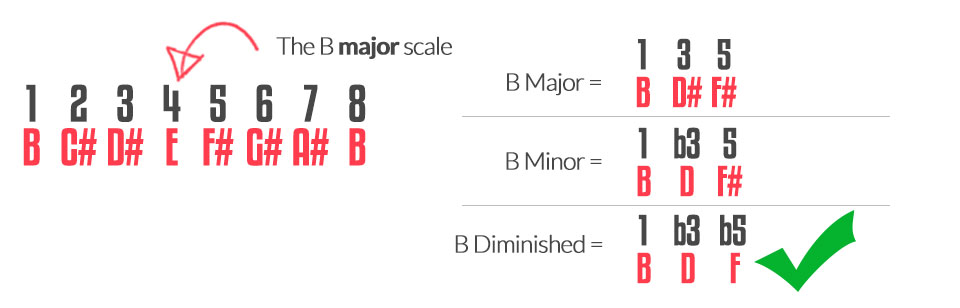So we can clearly see that the B diminished chord will fit into our C major scale as all 3 notes fit perfectly into our scale. The scale is C D E F G A B C, and in the B diminished chord you have a B, D & F. Perfect! Therefore the seventh chord of our key is B diminished.

Ready to move on? Remember to check out every lesson in this unit first – then try the next unit...

We will now bring together everything we have learnt so far and introduce the idea of relative minor scales. This is an important piece of theory as it is an extremely practical concept that will help turn all your major keys and scales into minor.